# Structural Vector Autoregression demo

This script illustrates how to work with Structural Vector Autoregression (SVAR) models with a simple example.
Results are produced using the VAR toolbox designed by Ambrogio Cesa-Bianchi, available at https://sites.google.com/site/ambropo/MatlabCodes.

## Model

The model is a structural VAR with three variables (Inflation, Output and Federal Funds Rate) and four lags.where variables are ranked in the following orderand with the following imposed restriction on the structural matrix A## Data

In this example, we estimate the model parameters on US data.
To construct the relevant variables, we use the following series from FRED (https://research.stlouisfed.org/fred2/):
• Consumer Price Index for All Urban Consumers: All Items (CPIAUCSL)
• Federal Funds Rate (FEDFUNDS)
• Civilian Noninstitutional Population (CNP16OV)
• Gross Domestic Product (GDP)
• Gross Domestic Product: Implicit Price Deflator (GDPDEF)
Here we use the Datafeed toolbox to fetch data from the FRED data server.
if contains(matlabpath,['toolbox' filesep 'datafeed'])
% Fetches data from FRED and saves it in raw_data.mat
try
% Create connection with FRED server
c = fred('https://research.stlouisfed.org/fred2/');
% Sample period
start_date = '01/01/1960';
end_date = '01/01/2019';
% Fetch data
rawdata.cpi = fetch(c, 'CPIAUCSL' ,start_date,end_date);
rawdata.ffr = fetch(c, 'FEDFUNDS' ,start_date,end_date);
rawdata.pop = fetch(c, 'CNP16OV' ,start_date,end_date);
rawdata.gdp = fetch(c, 'GDP' ,start_date,end_date);
rawdata.gdp_defl = fetch(c, 'GDPDEF' ,start_date,end_date);
% Save data
save raw_data rawdata start_date end_date
% Close connection with server
close(c); clearvars c
disp('Successfully fetched data!')
fetched = true;
catch
fetched = false;
end
else
disp('Could not find the datafeed toolbox.')
fetched = false;
end
Successfully fetched data!
if ~fetched
% Load raw data from .mat file
end

## Data preparation

Downloaded data has to be further manipulated before it can be used in our model.
In particular, we have to:
1. Convert monthly data (CPI, FEDFUNDS and CNP16OV) into quarterly data
2. Create a series for Inflation from the CPI index
3. Construct Real GDP per capita
4. Remove the trend component to get cyclical fluctuations of GDP

#### Monthly data to quarterly data

% Sample period
quarters = rawdata.gdp.Data(:,1);
% Quarters to months legend:
% Q1 --> 01-Jan
% Q2 --> 01-Apr
% Q3 --> 01-Jul
% Q4 --> 01-Oct
% To see dates, datestr(quarters)
% Monthly to quarterly data
rawdata.cpi.Data = rawdata.cpi.Data(ismember(rawdata.cpi.Data(:,1),quarters),:);
rawdata.ffr.Data = rawdata.ffr.Data(ismember(rawdata.ffr.Data(:,1),quarters),:);
rawdata.pop.Data = rawdata.pop.Data(ismember(rawdata.pop.Data(:,1),quarters),:);

#### Year-on-year inflation rate

T = length(quarters);
Pi = zeros(T-4,1);
for t = 5:T
Pi(t-4) = 100*(rawdata.cpi.Data(t,2) - rawdata.cpi.Data(t-4,2))/rawdata.cpi.Data(t-4,2);
end
% NOTE: The first four observations are lost when constructing Pi.
% Update sample period and data
quarters = quarters(5:end);
rawdata.gdp.Data = rawdata.gdp.Data(ismember(rawdata.gdp.Data(:,1),quarters),:);
rawdata.gdp_defl.Data = rawdata.gdp_defl.Data(ismember(rawdata.gdp_defl.Data(:,1),quarters),:);
rawdata.ffr.Data = rawdata.ffr.Data(ismember(rawdata.ffr.Data(:,1),quarters),:);
rawdata.pop.Data = rawdata.pop.Data(ismember(rawdata.pop.Data(:,1),quarters),:);

#### Real GDP per capita

% Create population index
index_date = '01-Jan-1990';
pop_index = rawdata.pop.Data(:,2) ./ rawdata.pop.Data(rawdata.pop.Data(:,1)==datenum(index_date),2);
% Deflate nominal GDP
gdp_real = rawdata.gdp.Data(:,2) ./ rawdata.gdp_defl.Data(:,2);
% Divide by population index
gdp_real_cap = gdp_real ./ pop_index;

#### Remove trend in output

% Choose detrending option: 'log-linear' or 'HP filtering'
detrend_opt = 'HP filtering';
switch detrend_opt
case 'log-linear'
Y = detrend(100.*log(gdp_real_cap));
case 'HP filtering'
[~,Y] = hpfilter(100.*log(gdp_real_cap),1600);
end

#### Cleaned data

dates = datetime(datestr(rawdata.ffr.Data(:,1)));
R = rawdata.ffr.Data(:,2);
% Final data ready to be used in estimation
data = timetable(dates,Pi,Y,R,'VariableNames',{'Inflation','Output','FedFunds'});
X = [Pi Y R];

#### Plot data (optional)

% Select 'true' or 'false' if you want to plot data
plot_data = true;
if plot_data
plot(dates,data.Variables)
legend(data.Properties.VariableNames)
end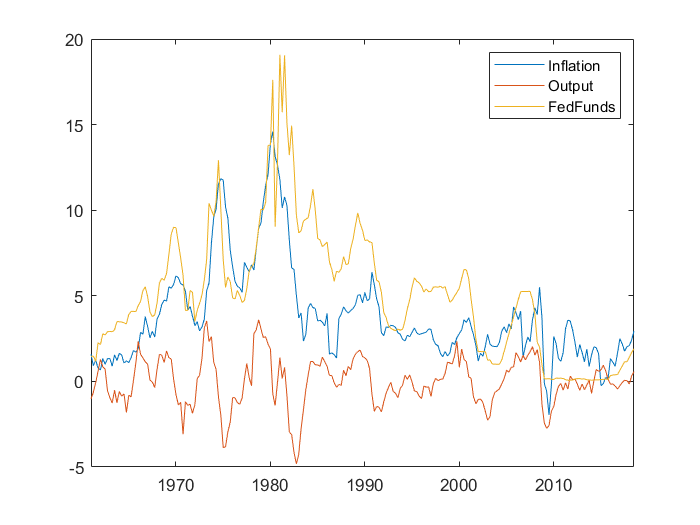## Estimation

Now that data is ready, we can estimate the VAR using the VAR Toolbox.
First, we need to tell MATLAB where the toolbox is located:
% Add VAR toolbox to the MATLAB path (including all subfolders)
Next, we need to specify some variables that determine the shape of our VAR, that is the number of lags and whether the VAR should have a constant, a trend or both.

#### Mean and trends

Before estimating the VAR, you need to choose between the following options:
• const_trend = 0 --> No constant and no trend
• const_trend = 1 --> Only constant and no trend
• const_trend = 2 --> Constant and trend
This choice depends on whether your endogenous variables (Inflation, Output and Interest rate) display a non-zero mean and a trend. Therefore, you should inspect your variables and make a choice accordingly.
In practice, it is recommended to include both constant and trend in your estimation. If it turns out that your time series does not have a non-zero mean nor a trend, the respective estimated parameters will be equal (or very close) to zero.
% Choose constant and trend
const_trend = 2;
% Choose number of lags
nbr_lags = 4;
Finally, call the appropriate function to estimate the model and show parameters estimates:
% Estimate the VAR
[VAR, VAR_options] = VARmodel(X,nbr_lags,const_trend);
% Show estimated model parameters
VAR_options.vnames = data.Properties.VariableNames;
VARprint(VAR,VAR_options);
Reduced form VAR estimation: Inflation Output FedFunds c 0.3964 0.2305 0.5102 trend -0.0011 -0.0007 -0.0026 Inflation(-1) 0.9528 -0.0588 -0.0015 Output(-1) 0.2775 1.0243 0.6967 FedFunds(-1) 0.0881 -0.0527 0.6162 Inflation(-2) -0.0356 0.0757 0.0148 Output(-2) -0.1874 0.0413 -0.2558 FedFunds(-2) 0.0713 -0.0060 0.1492 Inflation(-3) 0.1342 -0.0674 0.1320 Output(-3) -0.0946 -0.2336 -0.1915 FedFunds(-3) -0.0026 0.1522 0.2315 Inflation(-4) -0.1431 0.0373 -0.0364 Output(-4) 0.0965 -0.0337 0.0312 FedFunds(-4) -0.1396 -0.1135 -0.1190

## Impulse responses

In this section, we compute and plot the dynamic responses of endogenous variables to a structural shock.

#### Identification

But wait, what is a structural shock!? Remember in the slides we made an important distinction between innovations and structural shocks. Loosely speaking, a structural shock is a sudden movement in the error terms that we can clearly identify coming from a specific source. Conversely, innovations are sudden movements in the error terms which might be caused by several factors.
How do we tell a structural shock from an innovation? One way is to use economic theory to impose some restrictions on the parameters of the model. Given the restriction, we can now say that a sudden movement in the error term is due to a movement in a particular variable.
Remember when we introduced the model, we said that the structural matrix A was lower triangular. This is an example of one of such restrictions. This restriction implies that only some variables are able to contemporaneously influence the other variables. This identification scheme is called recursive or short-run restriction.
In particular, given the ordering of the variables and the restriction on A,we deduce that:
• The policy rate (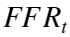) responds to contemporaneous changes to other variables
• Output (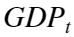) responds current inflation but not interest rate
• Inflation (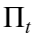) is not contemporaneously affected byorand only responds to these variables with a lag
This ordering of the variables is reasonable since we expect the central bank to immediately respond to movements in inflation and output with changes in the policy rate.
On the other hand, since both inflation and output are assumed to not contemporaneously react to movements in the policy rate, we can say that any sudden change in the error term ofis due to movements inonly. That is, it is a shock to the policy rate that does not come from a reaction to movements in other variables. This is a monetary policy shock.

#### Implementation

In practice, the restriction on the structural matrix A is imposed by doing a Cholesky decomposition of the estimated variance-covariance matrix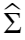of the residuals.
% Choose the identification scheme
VAR_options.ident = 'oir'; % 'oir' selects a recursive scheme
% Choose the horizon for the impulse responses
VAR_options.nsteps = 40;
% Apply the identification scheme and compute impulse responses
[IRF,VAR] = VARir(VAR,VAR_options);
It is usually good practice to report impulse responses along with confidence intervals.
The VAR toolbox computes a lower and an upper bound for the impulse responses via bootstrap:
1. The residuals are resampled and the model parameters are re-estimated a large number of times.
2. For each new estimate, impulse responses are computed.
3. All impulse responses are compared to identify the lower, upper and median impulse response.
% Compute confidence intervals using bootstrap methods
[IRF_lower,IRF_upper,IRF_median] = VARirband(VAR,VAR_options);
Loop 10 / 100 draws Loop 20 / 100 draws Loop 30 / 100 draws Loop 40 / 100 draws Loop 50 / 100 draws Loop 60 / 100 draws Loop 70 / 100 draws Loop 80 / 100 draws Loop 90 / 100 draws Loop 100 / 100 draws -- Done!
Finally, we can plot the impulse responses:
% Figures related options
VAR_options.savefigs = false;
VAR_options.quality = 0;
% Plot impulse response functions
VARirplot(IRF_median,VAR_options,IRF_lower,IRF_upper);
Impulse response to Inflation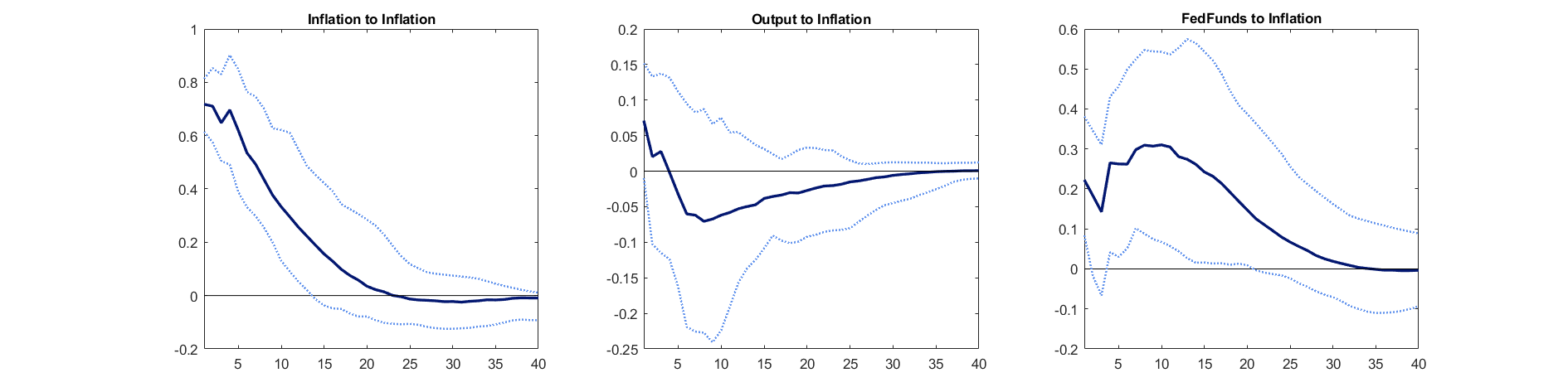Impulse response to Output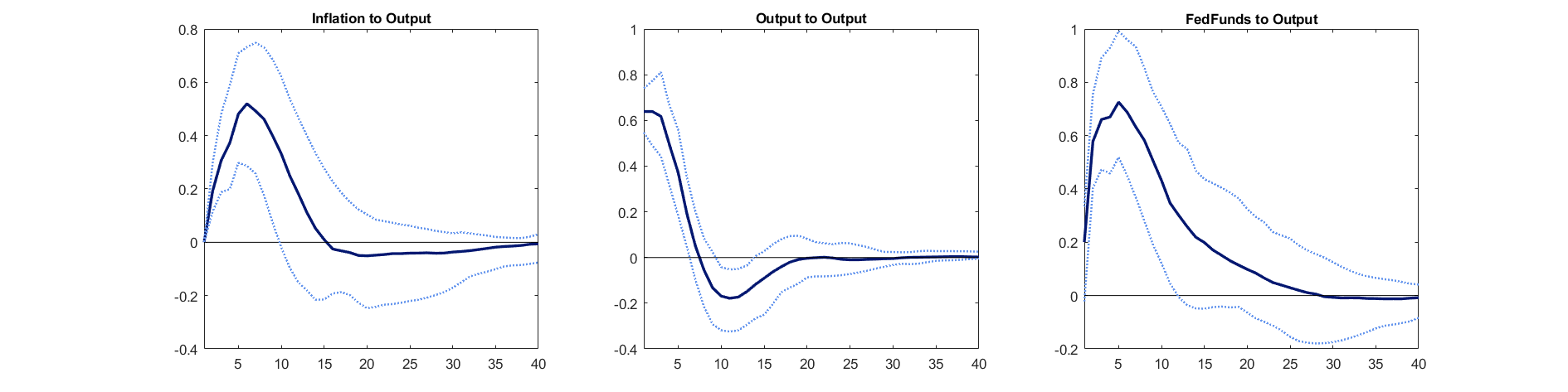Impulse response to FedFunds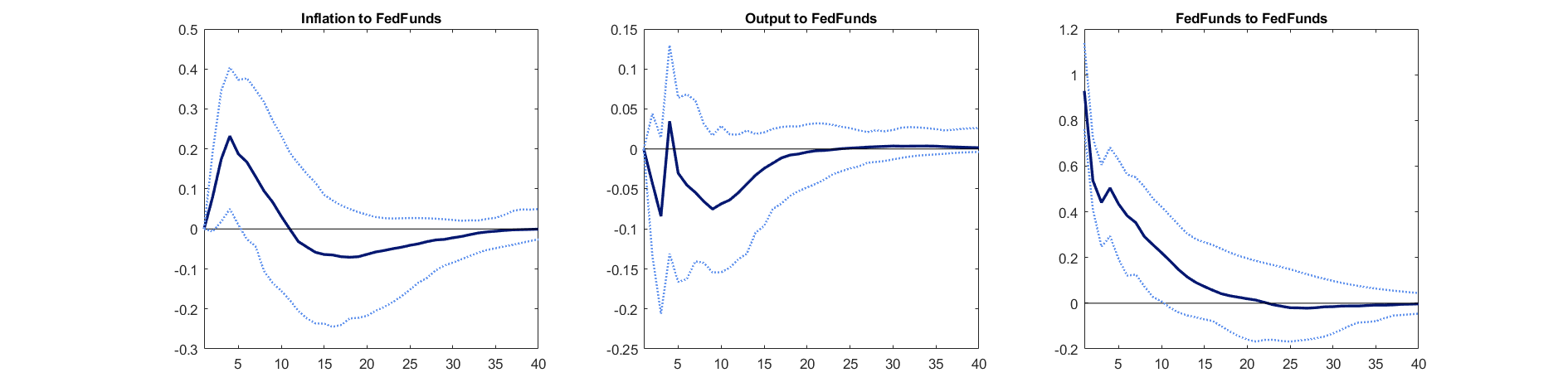## Historical decomposition

The historical decomposition summarises the history of each endogenous variable in the light of the VAR.
It asks the following question: given the estimated model, what is the sequence of shocks that is able to replicate the time series of Inflation, Output and Federal Funds Rate?
In other words, the historical decomposition tells us what portion of the deviation of the endogenous variables from their unconditional mean is due to the each shock.
% Compute historical decomposition
HistDecomp = VARhd(VAR);
% Plot historical decomposition
VARhdplot(HistDecomp,VAR_options);
Historical decomposition of Inflation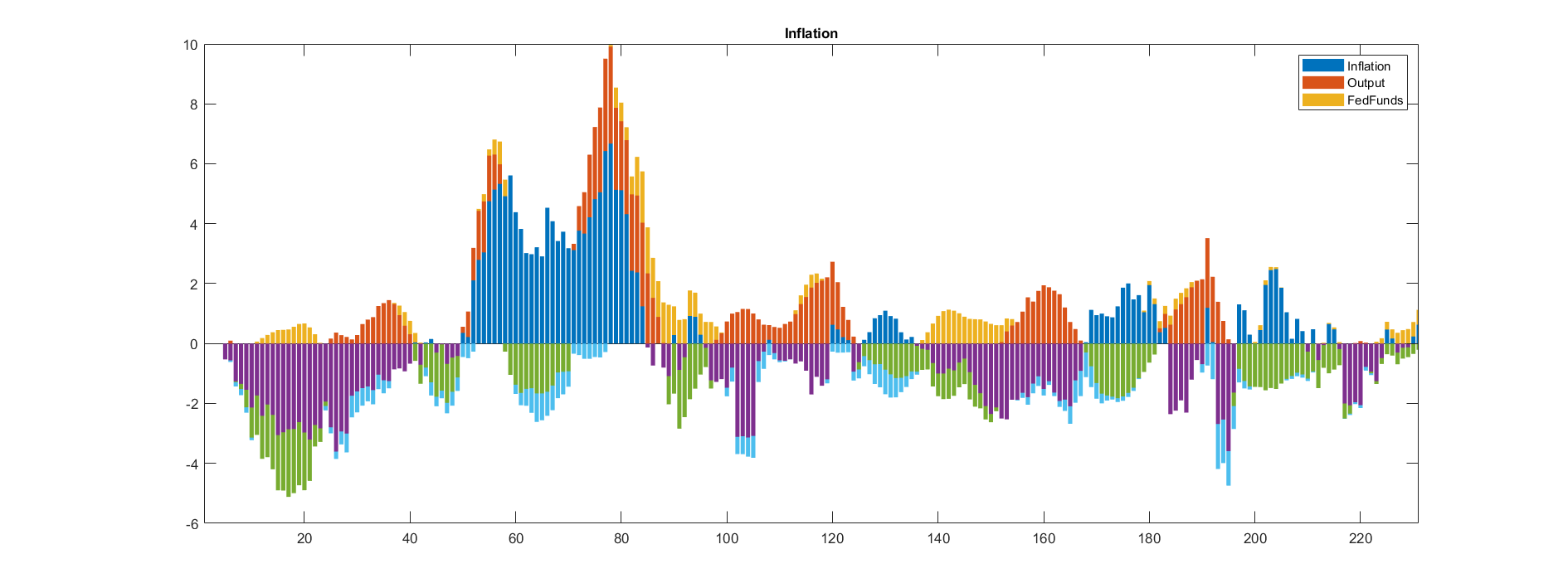Historical decomposition of Output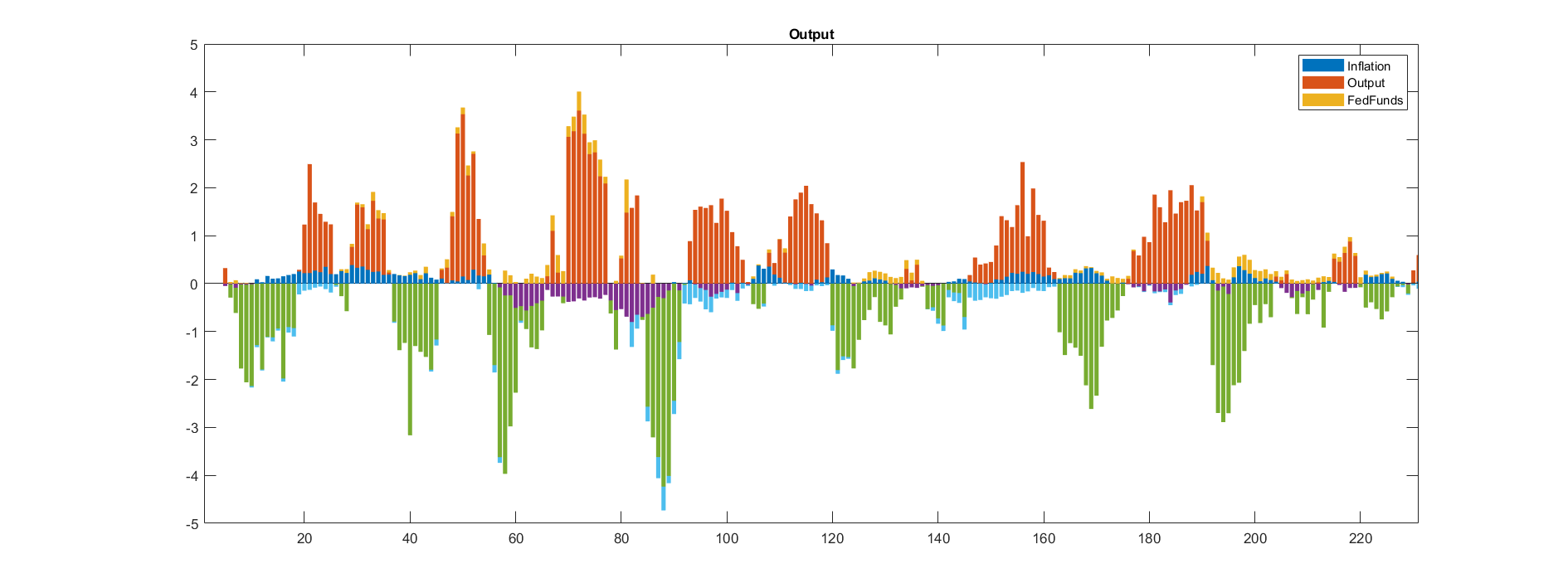Historical decomposition of FedFunds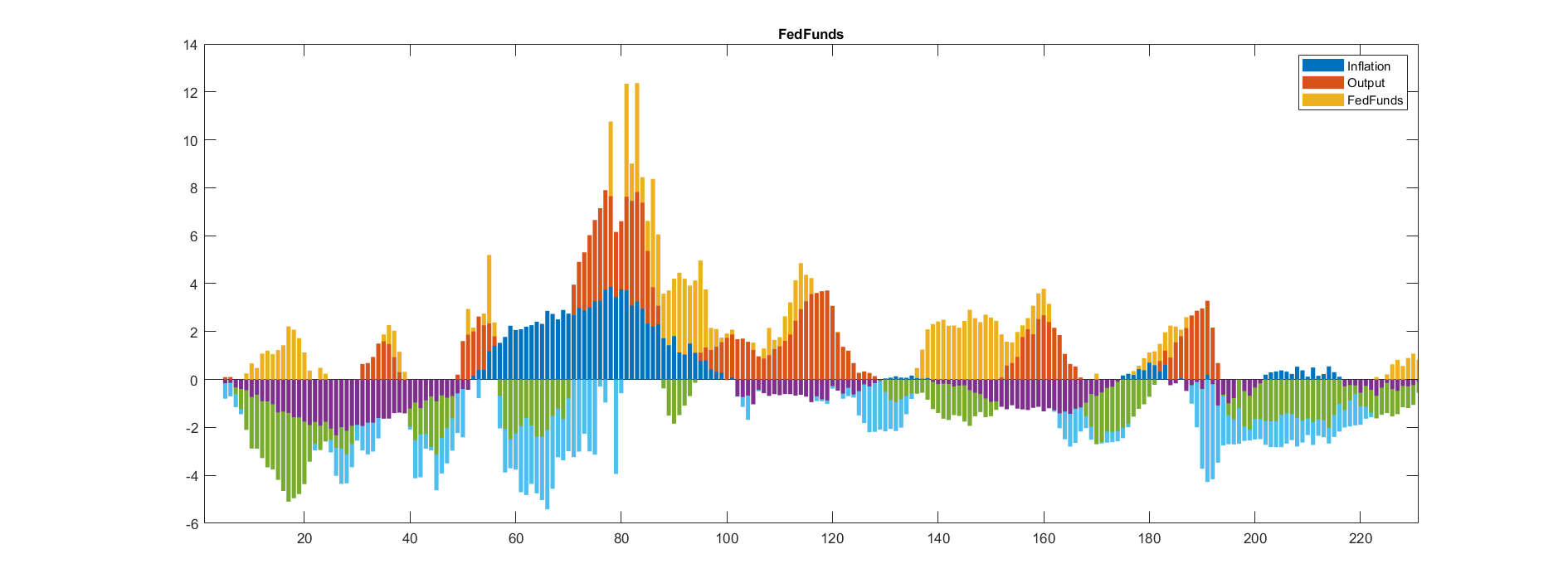## Forecast Error Variance decomposition

The variance decomposition of the forecast errors tells us the contribution of each shock to the total variability of each endogenous variable.
It is used to understand how much of the variability of each variable is explained by a given shock. The dynamic nature of the VAR model also implies that the contribution of each shock may change over time, for instance one shock may be important in the first few periods but less important in the long-run.
% Compute forecast error variance decomposition
[FEVD,VAR] = VARfevd(VAR,VAR_options);
% Compute confidence interval via bootstrap
[FEVDINF,FEVDSUP,FEVDMED] = VARfevdband(VAR,VAR_options);
Loop 10 / 100 draws Loop 20 / 100 draws Loop 30 / 100 draws Loop 40 / 100 draws Loop 50 / 100 draws Loop 60 / 100 draws Loop 70 / 100 draws Loop 80 / 100 draws Loop 90 / 100 draws Loop 100 / 100 draws -- Done!
% Plot
VARfevdplot(FEVDMED,VAR_options,FEVDINF,FEVDSUP);
Variance decomposition of Inflation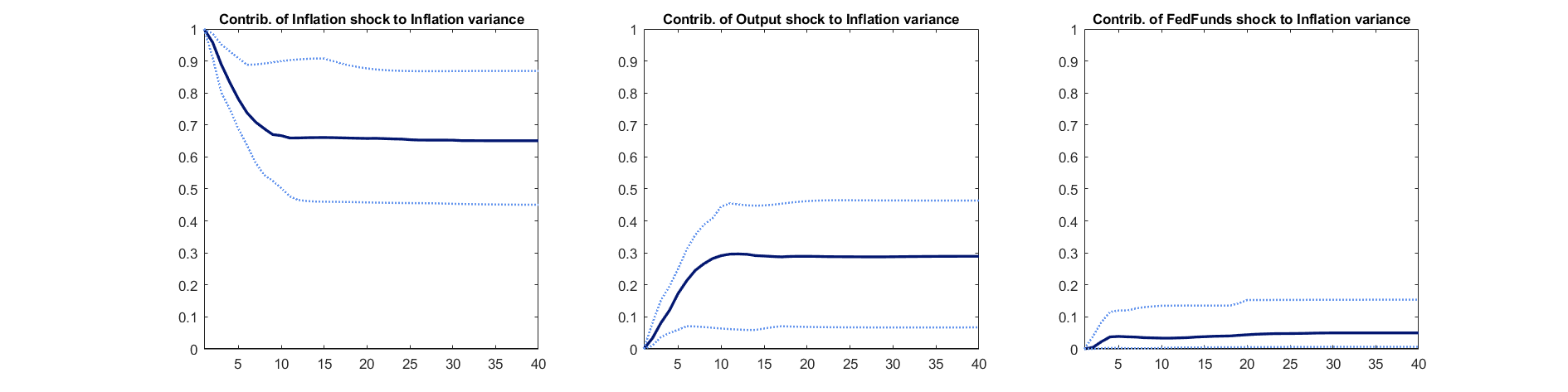Variance decomposition of Output